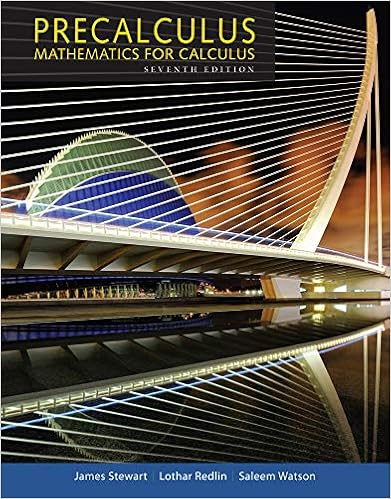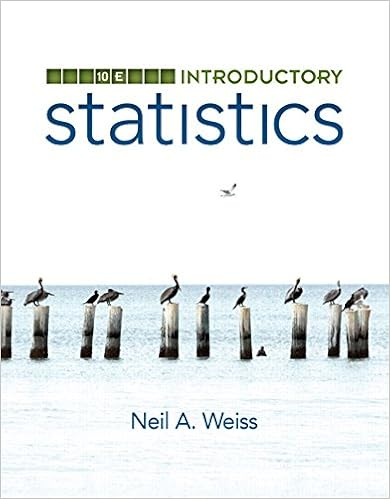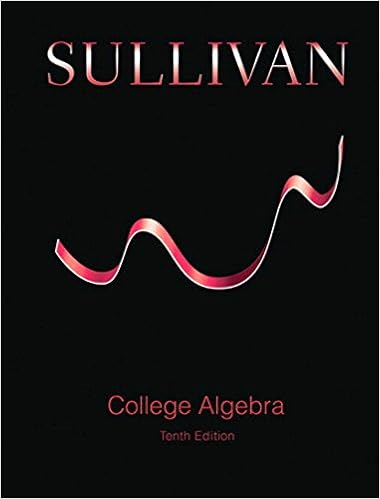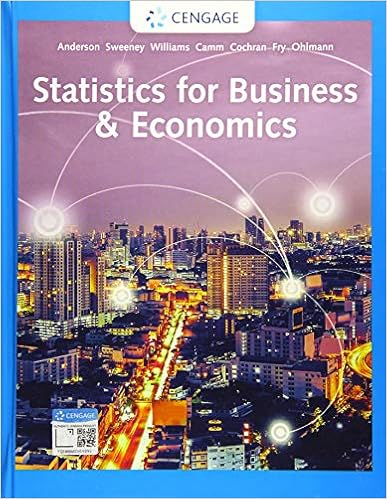# Problem Set 2 - Math 133, Practice Problems: Conics,...

• Homework Help
• HighnessDangerFish3844
• 2

This preview shows page 1 - 2 out of 2 pages.

The preview shows page 1 - 2 out of 2 pages.
Math 133, Practice Problems: Conics, Parametric Equations and Polar CoordinatesThe following questions are collected from some practice problems suggested to Math 122students when they study conics, polar coordinates and parametric equations.1.Write the equation of the conicx2+ 6x-8y-23 = 0 in standard form, and then findits vertex, focus and the equation of its directrix. (Think about similar questions for ellipsesand hyperbolas where you would find center, vertices, foci, and additionally asymptotes forhyperbolas)2.(a) Find the equation of the hyperbola with foci (2,-6) and (2,4) whose vertices are (2,-4)and (2,2); and find the equation of its asymptotes.(b) Find the equation of ellipse with vertices (-6,2) and (4,2) and foci (-4,2) and (2,2).(c) Sketch the conics you found in (a) and (b). (Think about analogous questions for parabolaswhere one is given, e.g. the focus and vertex).3.(a) Describe the graph whose polar equation isrsinθ= 3. Be specific!(b) Describe the graph whose polar equation isr= 4. Be specific!
##### We have textbook solutions for you!The document you are viewing contains questions related to this textbook.
Chapter 11 / Exercise 5
Precalculus: Mathematics for Calculus
Redlin/StewartExpert VerifiedBrowse all Textbook Solutions

Course Hero member to access this document

End of preview. Want to read all 2 pages?

Course Hero member to access this document

Term
Fall
Professor
JonD.Vanderwerff
Tags
Conic section, polar curves
##### We have textbook solutions for you!
The document you are viewing contains questions related to this textbook.The document you are viewing contains questions related to this textbook.
Chapter 11 / Exercise 5
Precalculus: Mathematics for Calculus
Redlin/StewartExpert Verified
•••